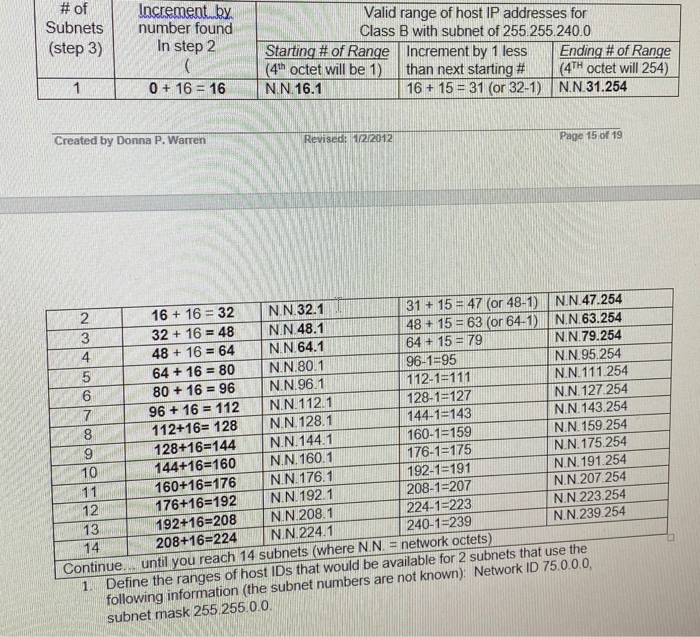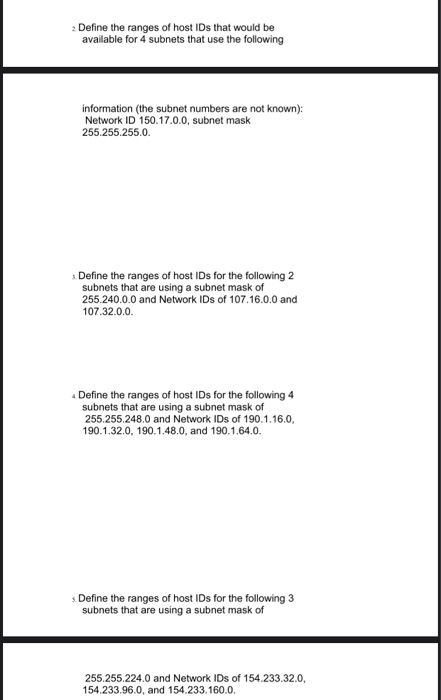# (Solved) : Subnets Step 3 Increment Number Found Step 2 Valid Range Host Ip Addresses Class B Subnet Q42745672 . . .to solve these questions# of Subnets (step 3) Increment by number found In step 2 Valid range of host IP addresses for Class B with subnet of 255.255.240.0 Starting # of Range Increment by 1 less Ending # of Range (4th octet will be 1) than next starting # (4TH octet will 254) N.N.16.1 16 + 15 = 31 (or 32-1) N.N.31.254 0 + 16 = 16 Created by Donna P. Warren Revised: 1/2/2012 Page 15 of 19 2 16 + 16 = 32 N.N.32.1 31 + 15 = 47 (or 48-1) N.N.47.254 32 + 16 = 48 N.N.48.1 148 + 15 = 63 (or 64-1 N.N.63.254 48 + 16 = 64 N.N.64.1 64 + 15 = 79 N.N.79.254 64 + 16 = 80 N.N.80.1 96-1=95 N.N.95.254 80 + 16 = 96 N.N.96.1 112-1=111 N.N.111.254 96 + 16 = 112 N.N.112.1 128-1=127 N.N.127.254 112+16= 128 NN 128.1 144-1=143 N.N. 143.254 128+16=144 N.N.144.1 160-1=159 N.N. 159.254 144+16=160 N.N. 160.1 N.N. 175.254 176-1=175 11 N.N.191.254 N.N. 176.1 160+16=176 192-1=191 NN 207 254 208-1=207 N.N. 1921 176+16=192 12 224-1=223 NN 223 254 NN 208.1 13 192+16=208 240-1=239 N.N. 224.1 NN.239.254 208+16=224 Continue… until you reach 14 subnets (where N N = network octets) 1. Define the ranges of host IDs that would be available for 2 subnets that use the following information (the subnet numbers are not known): Network ID 75.0.0.0, subnet mask 255 255.0.0. 14 T Define the ranges of host IDs that would be available for 4 subnets that use the following information (the subnet numbers are not known): Network ID 150.17.0.0, subnet mask 255.255.255.0. Define the ranges of host IDs for the following 2 subnets that are using a subnet mask of 255.240.0.0 and Network IDs of 107.16.0.0 and 107.32.0.0. Define the ranges of host IDs for the following 4 subnets that are using a subnet mask of 255.255.248.0 and Network IDs of 190.1.16.0, 190.1.32.0, 190.1.48.0, and 190.1.64.0. Define the ranges of host IDs for the following 3 subnets that are using a subnet mask of 255.255.224.0 and Network IDs of 154.233.32.0, 154.233.96.0, and 154.233.160.0. Show transcribed image text # of Subnets (step 3) Increment by number found In step 2 Valid range of host IP addresses for Class B with subnet of 255.255.240.0 Starting # of Range Increment by 1 less Ending # of Range (4th octet will be 1) than next starting # (4TH octet will 254) N.N.16.1 16 + 15 = 31 (or 32-1) N.N.31.254 0 + 16 = 16 Created by Donna P. Warren Revised: 1/2/2012 Page 15 of 19 2 16 + 16 = 32 N.N.32.1 31 + 15 = 47 (or 48-1) N.N.47.254 32 + 16 = 48 N.N.48.1 148 + 15 = 63 (or 64-1 N.N.63.254 48 + 16 = 64 N.N.64.1 64 + 15 = 79 N.N.79.254 64 + 16 = 80 N.N.80.1 96-1=95 N.N.95.254 80 + 16 = 96 N.N.96.1 112-1=111 N.N.111.254 96 + 16 = 112 N.N.112.1 128-1=127 N.N.127.254 112+16= 128 NN 128.1 144-1=143 N.N. 143.254 128+16=144 N.N.144.1 160-1=159 N.N. 159.254 144+16=160 N.N. 160.1 N.N. 175.254 176-1=175 11 N.N.191.254 N.N. 176.1 160+16=176 192-1=191 NN 207 254 208-1=207 N.N. 1921 176+16=192 12 224-1=223 NN 223 254 NN 208.1 13 192+16=208 240-1=239 N.N. 224.1 NN.239.254 208+16=224 Continue… until you reach 14 subnets (where N N = network octets) 1. Define the ranges of host IDs that would be available for 2 subnets that use the following information (the subnet numbers are not known): Network ID 75.0.0.0, subnet mask 255 255.0.0. 14 T
Define the ranges of host IDs that would be available for 4 subnets that use the following information (the subnet numbers are not known): Network ID 150.17.0.0, subnet mask 255.255.255.0. Define the ranges of host IDs for the following 2 subnets that are using a subnet mask of 255.240.0.0 and Network IDs of 107.16.0.0 and 107.32.0.0. Define the ranges of host IDs for the following 4 subnets that are using a subnet mask of 255.255.248.0 and Network IDs of 190.1.16.0, 190.1.32.0, 190.1.48.0, and 190.1.64.0. Define the ranges of host IDs for the following 3 subnets that are using a subnet mask of 255.255.224.0 and Network IDs of 154.233.32.0, 154.233.96.0, and 154.233.160.0.

Answer to # of Subnets (step 3) Increment by number found In step 2 Valid range of host IP addresses for Class B with subnet of 25…

We are the best freelance writing portal. Looking for online writing, editing or proofreading jobs? We have plenty of writing assignments to handle.